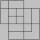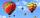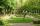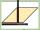# Right triangle - examples - page 23

1. SquareDanov's father has a square of 65.25 milligram square of wire with a diagonal. How will the square be big when one mm weighs 7 mg?
2. Cylinder horizontallyThe cylinder with a diameter of 3 m and a height/length of 15 m is laid horizontally. Water is poured into it, reaching a height of 60 cm below the axis of the cylinder. How many hectoliters of water is in the cylinder?
3. LadderLadder 10 meters long is staying against the wall so that its bottom edge is 6 meters away from the wall. What height reaches ladder?
4. Hot air balloonThe center of the balloon is at an altitude of 600 m above the ground (AGL). From habitat on earth is the center of the balloon to see in elevation angle 38°20' and the balloon is seen from the perspective of angle 1°16'. Calculate the diameter of the bal
5. PyramidThe pyramid has a base rectangle with a = 6cm, b = 8cm. The side edges are the same and their length = 12.5 cm. Calculate the surface of the pyramid.
6. Trapezoid - RRFind the area of the right angled trapezoid ABCD with the right angle at the A vertex; a = 3 dm b = 5 dm c = 6 dm d = 4 dm
7. Central park in cityThe city park has the shape of a rectangle of 180 meters in length and 120 meters in width. People make their walk through the center of the park from one corner to the second. Calculate how many meters this way is shorter than they walked along the path.
8. SquareCalculate the perimeter and the area of square with a diagonal length 30 cm.
9. Two aircraftTwo planes fly to the airport. At some point, the first airplane is away from the airport 98 km and the second 138 km. The first aircraft flies at an average speed of 420 km/h, the second average speed is 360 km/h, while the tracks of both planes are perpe
10. Chocolate rollThe cube of 5 cm chocolate roll weighs 30 g. How many calories will contain the same chocolate roller of a prism shape with a length of 0.5 m whose cross section is an isosceles trapezoid with bases 25 and 13 cm and legs 10 cm. You know that 100 g of this.
11. Pyramid four sidesIn a regular tetrahedral pyramid is a body height 38 cm and a wall height 42 cm. Calculate the surface area of the pyramid; the result round to square centimeters.
12. Quadrangular pyramidCalculate the surface area and volume of a regular quadrangular pyramid: sides of bases (bottom, top): a1 = 18 cm, a2 = 6cm angle α = 60 ° (Angle α is the angle between the side wall and the plane of the base.) S =? , V =?
13. Regular n-gonWhich regular polygon have a radius of circumscribed circle r = 10 cm and the radius of inscribed circle p = 9.962 cm?
14. Diagonals in diamons/rhombusRhombus ABCD has side length AB = 4 cm and a length of one diagonal of 6.4 cm. Calculate the length of the other diagonal.
15. Tree trunkFrom the tree trunk, the diameter at the narrower end is 28 cm, a beam of square cross-section is to be made. Calculate the longest side of the largest possible square cross-section.
16. Triangular prismThe perpendicular triangular prism is a right triangle with a 5 cm leg. The content of the largest wall of the prism is 130 cm2 and the body height is 10 cm. Calculate the body volume.
17. Rhombus OWESOWES is a rhombus given that OW 6cm and one diagonal measures 8cm. Find its area?
18. HypotenuseCalculate the length of the hypotenuse of a right triangle if the length of one leg is 4 cm and its content area is 16 square centimeters.
19. ConeCalculate the volume of the rotating cone with a base radius 26.3 cm and a side 38.4 cm long.
20. RT perimeterThe leg of the rectangular triangle is 7 cm shorter than the second leg and 8 cm shorter than the hypotenuse. Calculate the triangle circumference.

Do you have an interesting mathematical example that you can't solve it? Enter it, and we can try to solve it.

To this e-mail address, we will reply solution; solved examples are also published here. Please enter e-mail correctly and check whether you don't have a full mailbox.

See also our right triangle calculator.